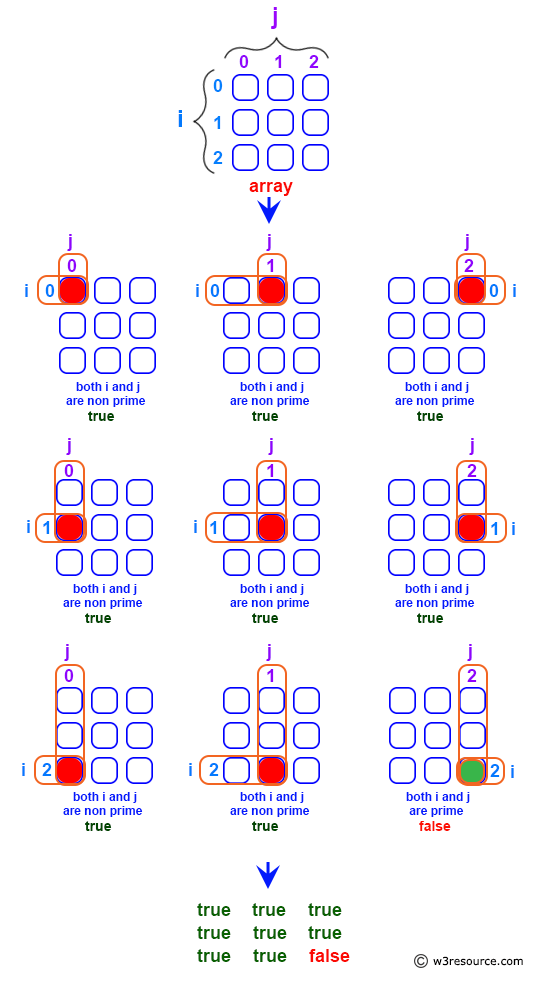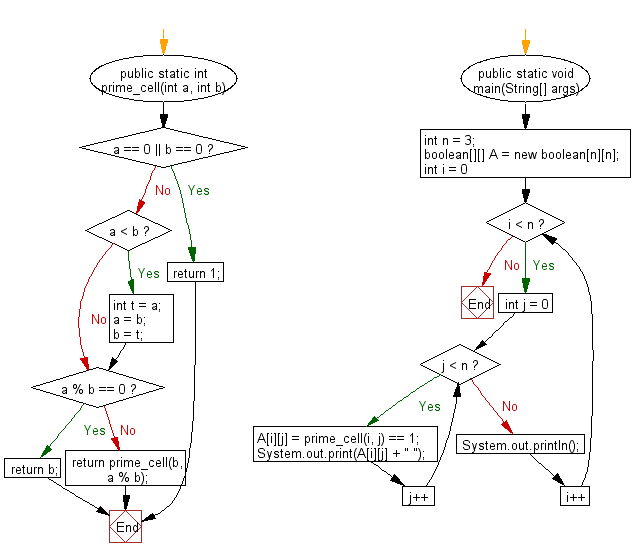﻿ Java - Create a 2-D array such that A[i][j] is false if I and j are prime, otherwise true

# Java: Create a 2-D array such that A[i][j] is false if I and j are prime, otherwise true

## Java Basic: Exercise-158 with Solution

Write a Java program to create a two-dimensional array (m x m) A[][] such that A[i][j] is false if I and j are prime otherwise A[i][j] becomes true.

Pictorial Presentation:Sample Solution:

Java Code:

``````import java.util.Scanner;
public class Solution {
public static int prime_cell(int a, int b) {
if (a == 0 || b == 0) {
return 1;
}
if (a < b) {
int t = a;
a = b;
b = t;
}
if (a % b == 0)
{
return b;
}
else
{
return prime_cell(b, a % b);
}
}

public static void main(String[] args) {
int n = 3;
boolean[][] A = new boolean[n][n];
for (int i = 0; i < n; i++) {
for (int j = 0; j < n; j++) {
A[i][j] = prime_cell(i, j) == 1;
System.out.print(A[i][j] + " ");
}
System.out.println();
}
}
}
```
```

Sample Output:

```true true true
true true true
true true false
```

Flowchart:Java Code Editor: